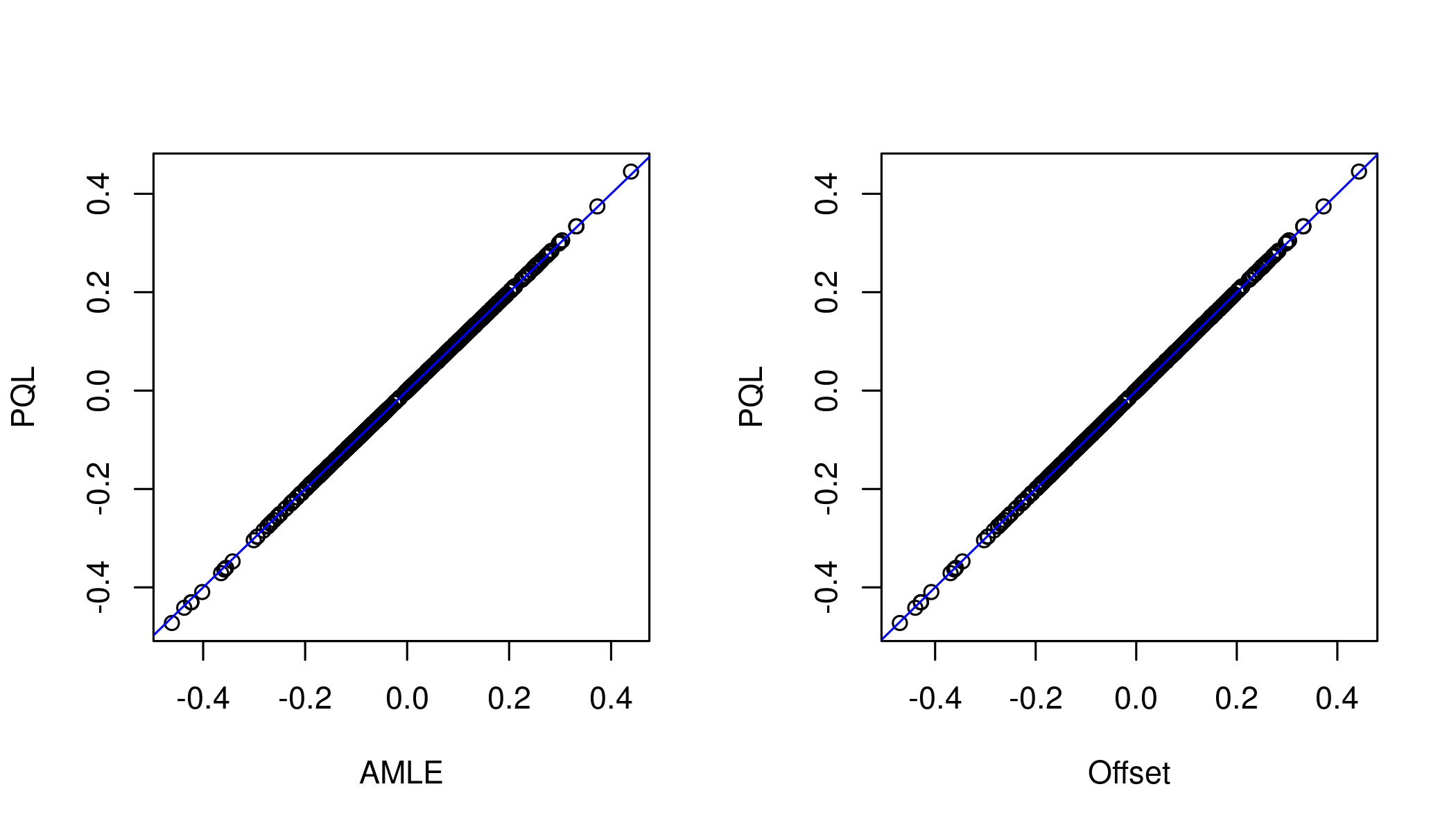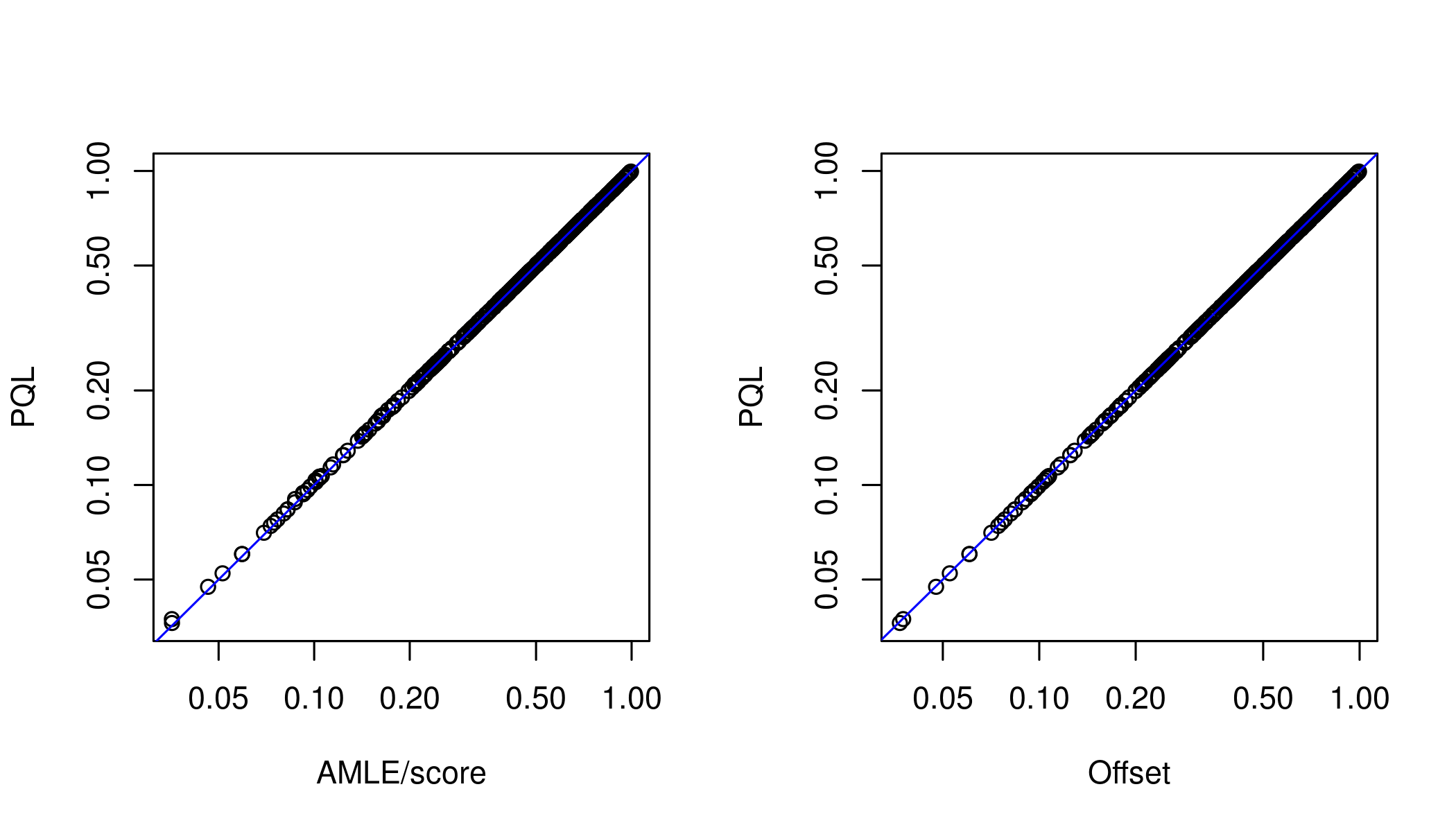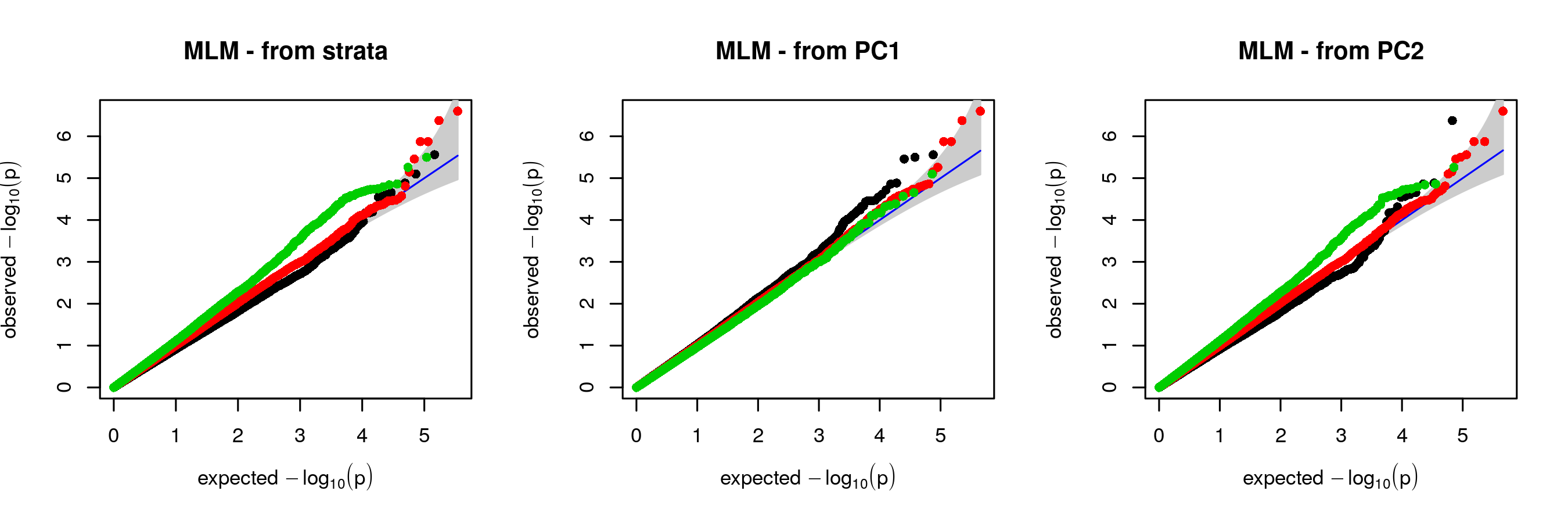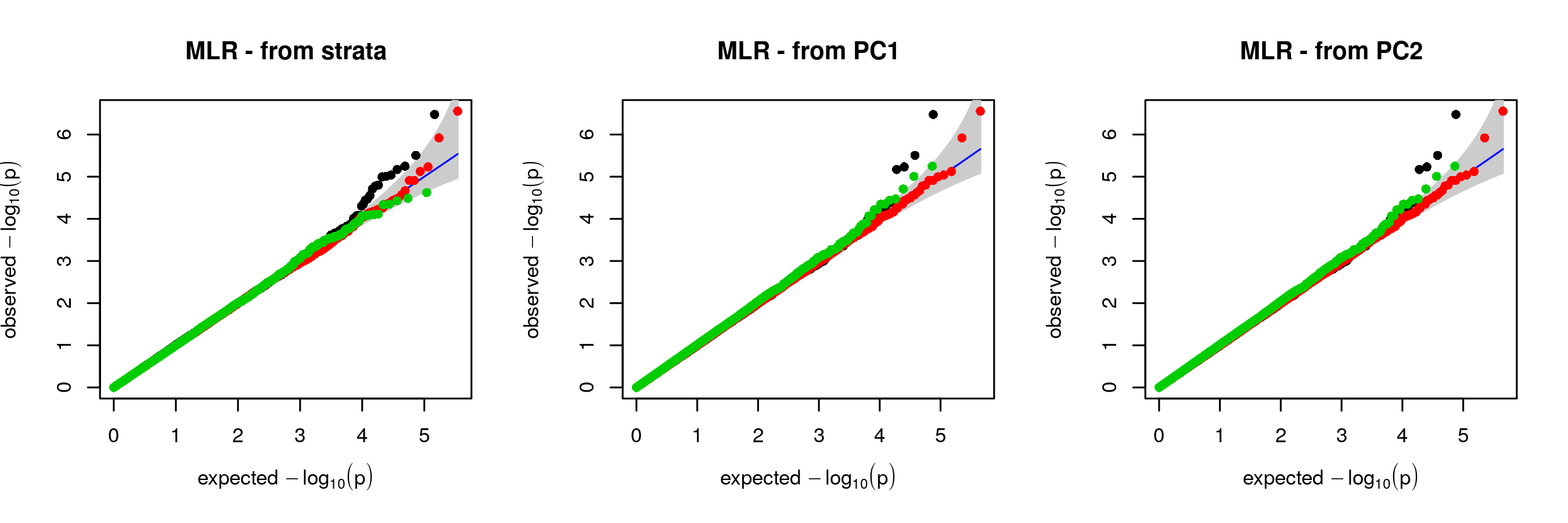# Introduction

This package is for Genome Wide Association Studies using a logistic mixed model, using fast approximate methods as described in (Milet and Perdry, 2020). One of these methods extends the GMMAT method by Chen et al. (Chen et al., 2016). A similar method was described in (Zhou et al., 2020).

Additionnally, it can draw QQ-plots with a separation of SNPs in strata, as defined by Chen et al. and extended in Milet and Perdry.

This package relies on the package gaston, which we will use in the following. We are going to illustrate below the functions exported by milorGWAS: association.test.logistic, qqplot.pvalues (which replaces and extends the function gaston::qqplot.pvalues), and SNP.category.

# Running association.test.logistic

## Building a small data set

In the following code, after loading the package, we use an example from gaston to simulate a binary phenotype with a random component $$\omega \sim N(0, \tau K)$$, in the model $\text{logit} P(Y = 1) = X \beta + \omega.$

The following lines creates a small genotype matrix x containing data from 1000 genomes, for 503 europeans and 733 SNPs on a region including the TTN gene (?TTN for details). The simulation of the phenotype can be done as follows.

library(milorGWAS)
x <- as.bed.matrix(TTN.gen, TTN.fam, TTN.bim)
x
## A bed.matrix with 503 individuals and 733 markers.
## snps stats are set
## ped stats are set
options(gaston.verbose = FALSE)

Let’s simulate a random phenotype.

set.seed(1)
## some covariates : an intercept, and a uniformly distributed covariate.
X <- cbind(1, runif(nrow(x)))
## A random GRM
ran <- random.pm(nrow(x))
## random effects (with variance tau = 1)
omega <- lmm.simu(1, 0, eigenK=ran$eigen)$omega
## linear term of the model
L <- X %*% c(0.1,-0.2) + omega
## vector of probabilities p = expit(L)
p <- 1/(1+exp( -L ))
## vector of binary phenotypes
y <- rbinom(length(p), 1, p)

## Logistic mixed model with Gaston

The package gaston can analyze these data with the Penalized Quasi Likelihood method (a0 below), which is too computationnaly heavy to scale to a GWAS, or with a score test, similar to GMMAT (Chen et al., 2016) (a1 below), which is fast but does not estimate $$\beta$$’s for each SNP.

a.pql <- association.test(x, y, X, K = ran$K, method = "lmm", response = "bin", test = "wald") a.gmm <- association.test(x, y, X, K = ran$K, method = "lmm", response = "bin", test = "score")

Here are the results for the first 6 SNPs of the data set, which give similar $$p$$-values.

head(a.pql)
##   chr       pos         id A1 A2    freqA2        tau        beta        sd          p
## 1   2 179200322  rs7571247  C  T 0.9025845 0.02676486 -0.36346036 0.2172089 0.09426397
## 2   2 179200714  rs3813253  G  A 0.7664016 0.02507730  0.04752710 0.1525129 0.75532412
## 3   2 179200947  rs6760059  T  C 0.8399602 0.02617219 -0.08552013 0.1760519 0.62713257
## 4   2 179201048 rs16866263  T  G 0.9433400 0.02588978  0.15828800 0.2729198 0.56192813
## 5   2 179201380 rs77946091  A  G 0.9433400 0.02589061  0.15828758 0.2729198 0.56192916
## 6   2 179201557 rs77711640  A  G 0.9423459 0.02560713  0.12033790 0.2707140 0.65666641
head(a.gmm)
##   chr       pos         id A1 A2    freqA2      score          p
## 1   2 179200322  rs7571247  C  T 0.9025845 2.83461240 0.09225306
## 2   2 179200714  rs3813253  G  A 0.7664016 0.09741459 0.75495457
## 3   2 179200947  rs6760059  T  C 0.8399602 0.23637475 0.62683681
## 4   2 179201048 rs16866263  T  G 0.9433400 0.33748981 0.56128175
## 5   2 179201380 rs77946091  A  G 0.9433400 0.33748981 0.56128175
## 6   2 179201557 rs77711640  A  G 0.9423459 0.19810102 0.65625803

## Logistic mixed model with milorGWAS

MilorGWAS proposes two more methods, named offset and amle (Milet and Perdry, 2020, for details). Again we show the results for the six first SNPs.

a.ofst <- association.test.logistic(x, y, X, K = ran$K, algorithm = "offset") a.amle <- association.test.logistic(x, y, X, K = ran$K, algorithm = "amle")
head(a.ofst)
##   chr       pos         id A1 A2    freqA2        beta        sd          p
## 1   2 179200322  rs7571247  C  T 0.9025845 -0.36176887 0.2164701 0.09467853
## 2   2 179200714  rs3813253  G  A 0.7664016  0.04734463 0.1520347 0.75549147
## 3   2 179200947  rs6760059  T  C 0.8399602 -0.08510584 0.1754785 0.62768126
## 4   2 179201048 rs16866263  T  G 0.9433400  0.15752648 0.2720386 0.56254914
## 5   2 179201380 rs77946091  A  G 0.9433400  0.15752648 0.2720386 0.56254914
## 6   2 179201557 rs77711640  A  G 0.9423459  0.11977405 0.2698536 0.65715228
head(a.amle)
##   chr       pos         id A1 A2    freqA2        beta        sd          p
## 1   2 179200322  rs7571247  C  T 0.9025845 -0.35834443 0.2128403 0.09225306
## 2   2 179200714  rs3813253  G  A 0.7664016  0.04755516 0.1523651 0.75495457
## 3   2 179200947  rs6760059  T  C 0.8399602 -0.08537859 0.1756097 0.62683681
## 4   2 179201048 rs16866263  T  G 0.9433400  0.15812891 0.2721955 0.56128175
## 5   2 179201380 rs77946091  A  G 0.9433400  0.15812891 0.2721955 0.56128175
## 6   2 179201557 rs77711640  A  G 0.9423459  0.12029441 0.2702726 0.65625803

The $$p$$-value from the amle method is always the same as the $$p$$-value from the score test (GMMAT).

## Comparing the results

We can compare the $$\beta$$’s obtained by the amle or offset algorithm with the values obtained with the PQL:

par(mfrow=c(1,2), cex = 0.9)
plot(a.amle$beta, a.pql$beta, xlab = "AMLE", ylab = "PQL"); abline(0,1,col=4)
plot(a.ofst$beta, a.pql$beta, xlab = "Offset", ylab = "PQL"); abline(0,1,col=4)A similar comparison for the $$p$$-values (remember that amle gives the same $$p$$-values than the score test).

par(mfrow=c(1,2), cex = 0.9)
plot(a.amle$p, a.pql$p, log = "xy", xlab = "AMLE/score", ylab = "PQL"); abline(0,1,col=4)
plot(a.ofst$p, a.pql$p, log = "xy", xlab = "Offset", ylab = "PQL"); abline(0,1,col=4)# Stratified qq-plots

Here we will use a simulated structured population with a large number of SNPs to illustrate the stratified qq-plots. These data simulated as described in (Chen et al., 2016) or in (Milet and Perdry, 2020) with the program ms (Hudson, 2002). We created a data package for these data. It includes 600k SNPs at linkage equilibrium for 1000 individuals simulated on a 20x20 grid. It includes some first-order related individuals. A binary phenotype was simulated with a large population structure effect as described in (Milet and Perdry, 2020).

The package needs to be installed first.

install.packages("GridData", repos="https://genostats.github.io/R/")

Then the data are easily loaded with

filepath <-system.file("extdata", "GridData.bed", package="GridData")
x <- set.stats(x)

A look at the data size:

x
## A bed.matrix with 1000 individuals and 600000 markers.
## snps stats are set
## ped stats are set

At the two population strata, which are included in the x@ped data frame:

table(x@ped$pop) ## ## 0 1 ## 768 232 At the simulated phenotype: table(x@ped$pheno)
##
##   0   1
## 877 123

And finally at the relation between strata and phenotype

table(x@ped$pop, x@ped$pheno)
##
##       0   1
##   0 714  54
##   1 163  69

## Running the association test

The GRM is computed with Gaston, here on the whole set of SNPs (at linkage equilibrium).

K <- GRM(x)
eigenK <- eigen(K)

We run a Mixed Linear Model (MLM) and a Mixed Logistic Regression (MLR).

MLM <- association.test(x, method = "lmm", response = "quantitative",
test = "wald", eigenK = eigenK, p = 10)
MLR <- association.test.logistic(x,  K = K, eigenK = eigenK, p = 10, algorithm = "amle")

## Drawing stratified QQ-plots

Now we can draw stratified quantile-quantile plots. We need to defined SNP categories, which can be done either with the ‘true’ strata information as described in (Chen et al.,2016), or with the first PCs (Milet and Perdry, 2020).

The function SNP.category can handle any kind of variable: strata information, or a Principal Component as a proxy.

# SNPs categories from true strata information
cat.str <- SNP.category(x,x@ped$pop) # SNPs categories from the first PCs coordinates cat.PC1 <- SNP.category(x,-eigenK$vectors[,1])
cat.PC2 <- SNP.category(x,-eigenK\$vectors[,2])

First, the QQ-plots obtained with the mixed linear model, which shows differences between SNP categories:

par(mfrow=c(1,3), cex = 0.7)
qqplot.pvalues(MLM, cat.str, col.abline="blue", main="MLM - from strata", pch = 16)
qqplot.pvalues(MLM, cat.PC1, col.abline="blue", main="MLM - from PC1", pch = 16)
qqplot.pvalues(MLM, cat.PC2, col.abline="blue", main="MLM - from PC2", pch = 16)Then, the QQ-plot obtained with mixed logistic regression, in which no such differences exist.

par(mfrow=c(1,3), cex = 0.7)
qqplot.pvalues(MLR, cat.str, col.abline="blue", main="MLR - from strata", pch = 16)
qqplot.pvalues(MLR, cat.PC1, col.abline="blue", main="MLR - from PC1", pch = 16)
qqplot.pvalues(MLR, cat.PC1, col.abline="blue", main="MLR - from PC2", pch = 16)